Скачать презентацию Chapter 17 The Flow of Energy Energy

• Количество слайдов: 67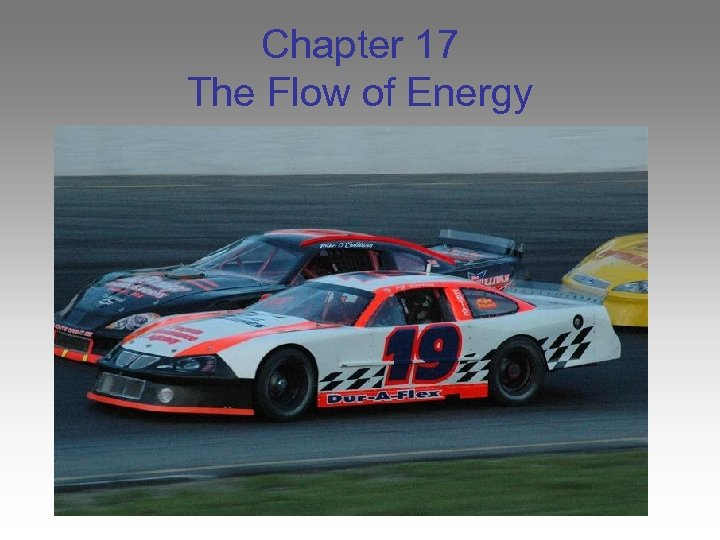Chapter 17 The Flow of Energy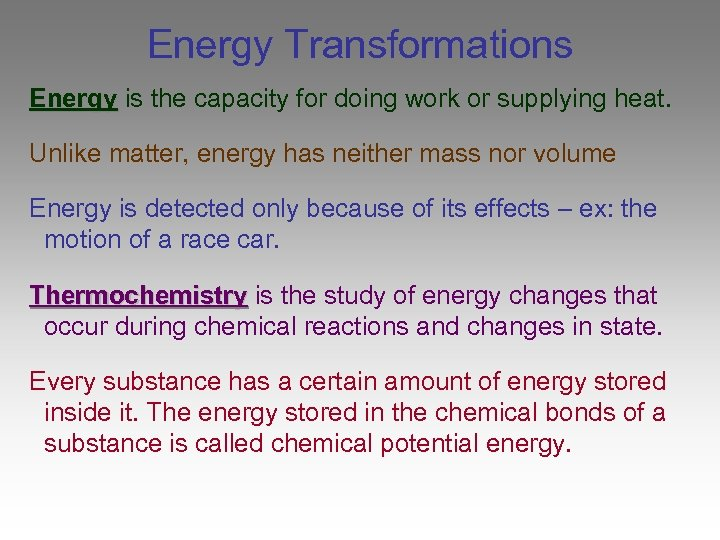Energy Transformations Energy is the capacity for doing work or supplying heat. Unlike matter, energy has neither mass nor volume Energy is detected only because of its effects – ex: the motion of a race car. Thermochemistry is the study of energy changes that occur during chemical reactions and changes in state. Every substance has a certain amount of energy stored inside it. The energy stored in the chemical bonds of a substance is called chemical potential energy.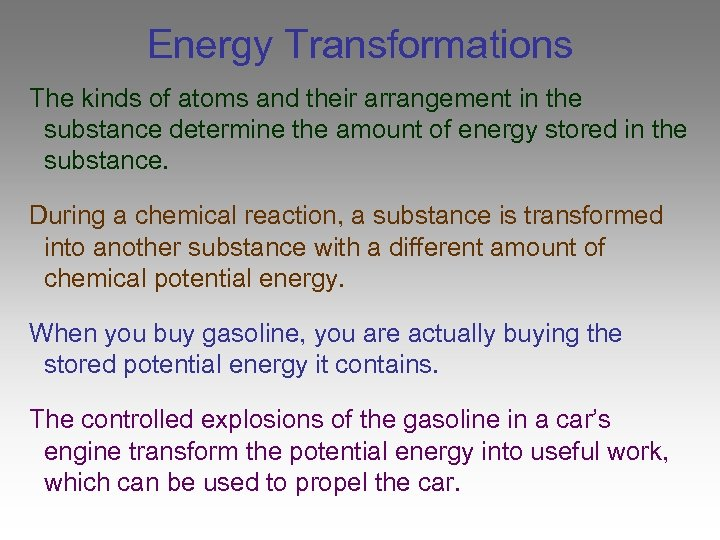Energy Transformations The kinds of atoms and their arrangement in the substance determine the amount of energy stored in the substance. During a chemical reaction, a substance is transformed into another substance with a different amount of chemical potential energy. When you buy gasoline, you are actually buying the stored potential energy it contains. The controlled explosions of the gasoline in a car’s engine transform the potential energy into useful work, which can be used to propel the car.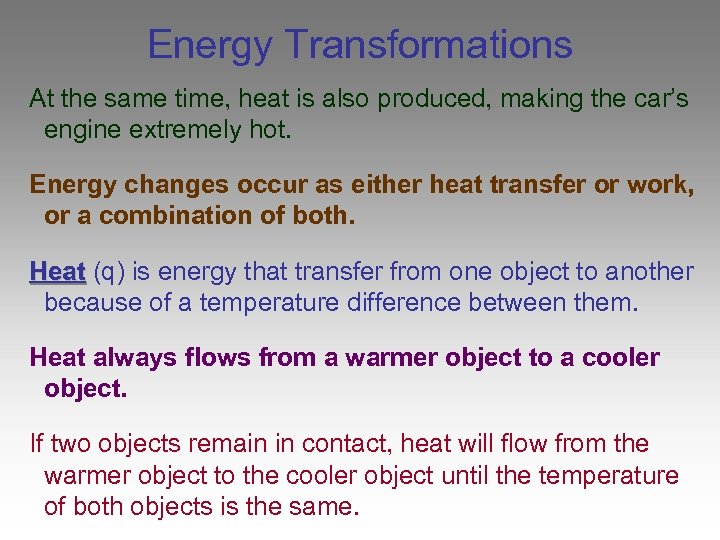Energy Transformations At the same time, heat is also produced, making the car’s engine extremely hot. Energy changes occur as either heat transfer or work, or a combination of both. Heat (q) is energy that transfer from one object to another because of a temperature difference between them. Heat always flows from a warmer object to a cooler object. If two objects remain in contact, heat will flow from the warmer object to the cooler object until the temperature of both objects is the same.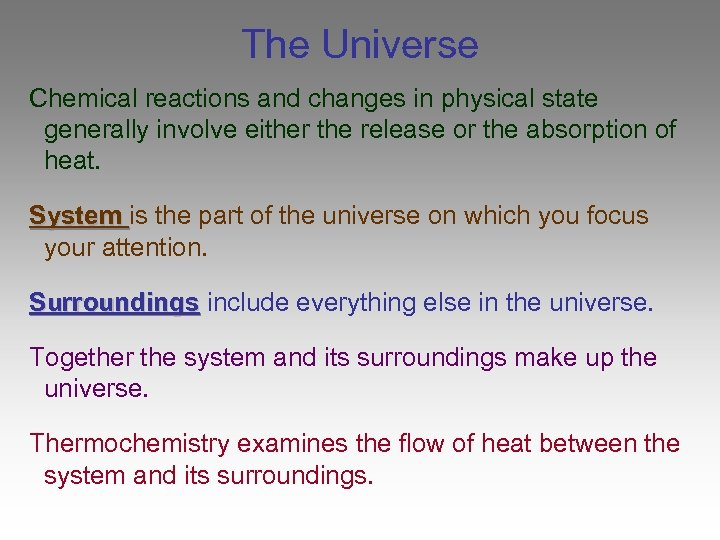The Universe Chemical reactions and changes in physical state generally involve either the release or the absorption of heat. System is the part of the universe on which you focus your attention. Surroundings include everything else in the universe. Together the system and its surroundings make up the universe. Thermochemistry examines the flow of heat between the system and its surroundings.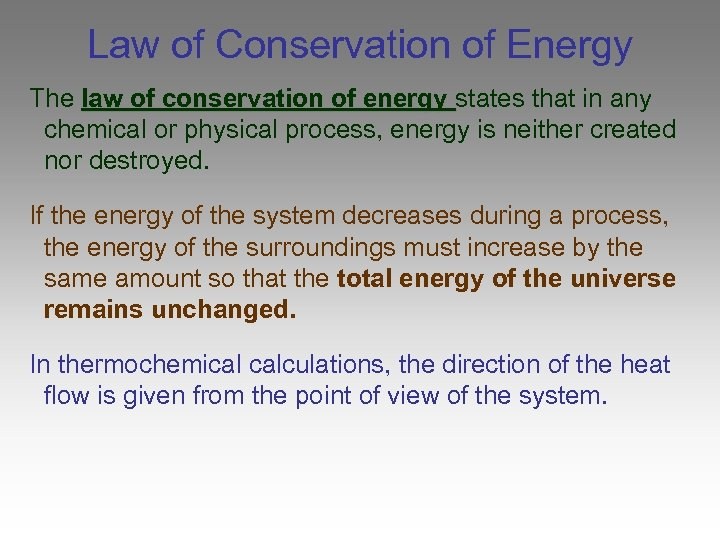Law of Conservation of Energy The law of conservation of energy states that in any chemical or physical process, energy is neither created nor destroyed. If the energy of the system decreases during a process, the energy of the surroundings must increase by the same amount so that the total energy of the universe remains unchanged. In thermochemical calculations, the direction of the heat flow is given from the point of view of the system.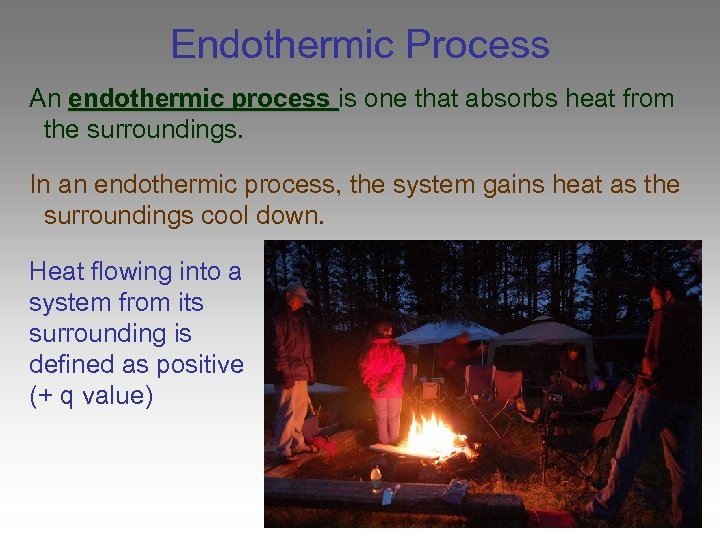Endothermic Process An endothermic process is one that absorbs heat from the surroundings. In an endothermic process, the system gains heat as the surroundings cool down. Heat flowing into a system from its surrounding is defined as positive (+ q value)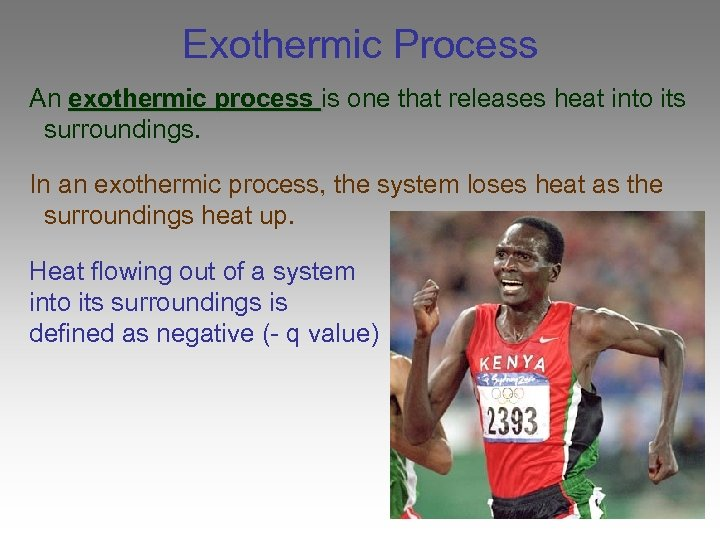Exothermic Process An exothermic process is one that releases heat into its surroundings. In an exothermic process, the system loses heat as the surroundings heat up. Heat flowing out of a system into its surroundings is defined as negative (- q value)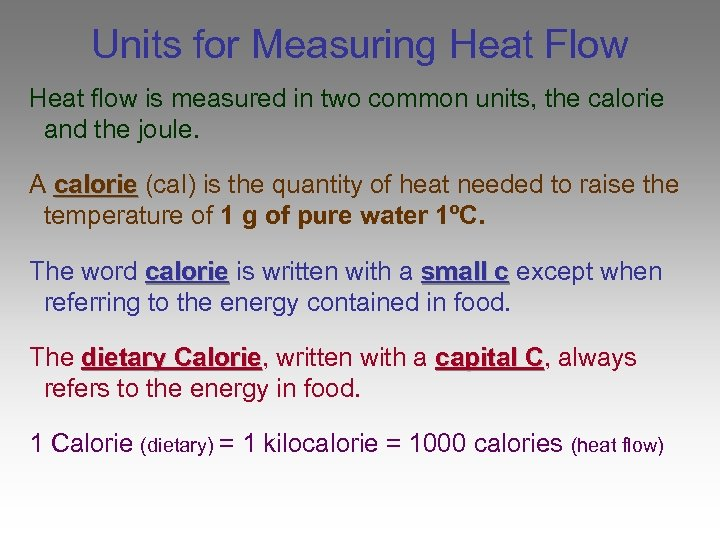Units for Measuring Heat Flow Heat flow is measured in two common units, the calorie and the joule. A calorie (cal) is the quantity of heat needed to raise the temperature of 1 g of pure water 1ºC. The word calorie is written with a small c except when referring to the energy contained in food. The dietary Calorie, written with a capital C, always Calorie C refers to the energy in food. 1 Calorie (dietary) = 1 kilocalorie = 1000 calories (heat flow)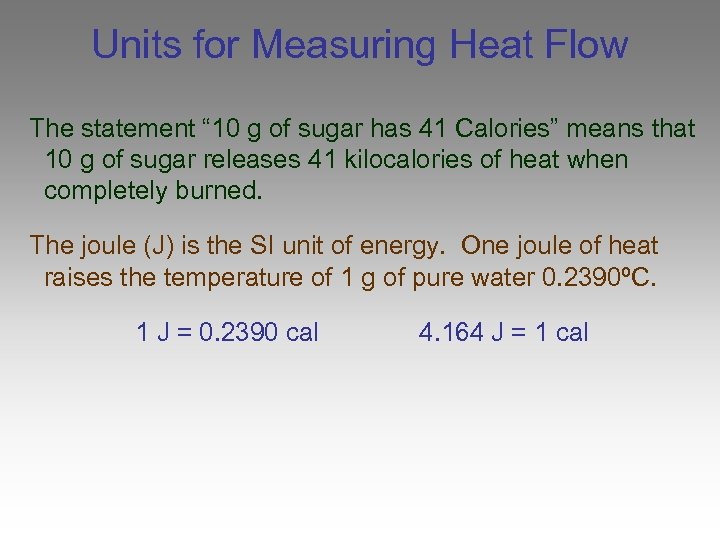Units for Measuring Heat Flow The statement “ 10 g of sugar has 41 Calories” means that 10 g of sugar releases 41 kilocalories of heat when completely burned. The joule (J) is the SI unit of energy. One joule of heat raises the temperature of 1 g of pure water 0. 2390ºC. 1 J = 0. 2390 cal 4. 164 J = 1 cal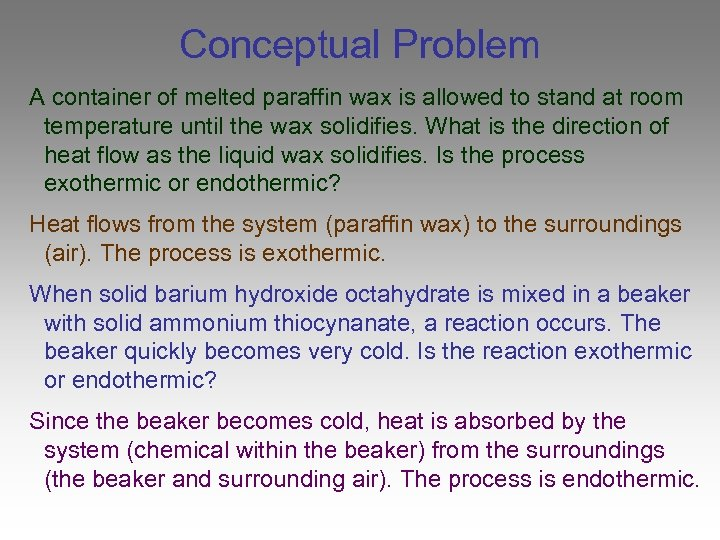Conceptual Problem A container of melted paraffin wax is allowed to stand at room temperature until the wax solidifies. What is the direction of heat flow as the liquid wax solidifies. Is the process exothermic or endothermic? Heat flows from the system (paraffin wax) to the surroundings (air). The process is exothermic. When solid barium hydroxide octahydrate is mixed in a beaker with solid ammonium thiocynanate, a reaction occurs. The beaker quickly becomes very cold. Is the reaction exothermic or endothermic? Since the beaker becomes cold, heat is absorbed by the system (chemical within the beaker) from the surroundings (the beaker and surrounding air). The process is endothermic.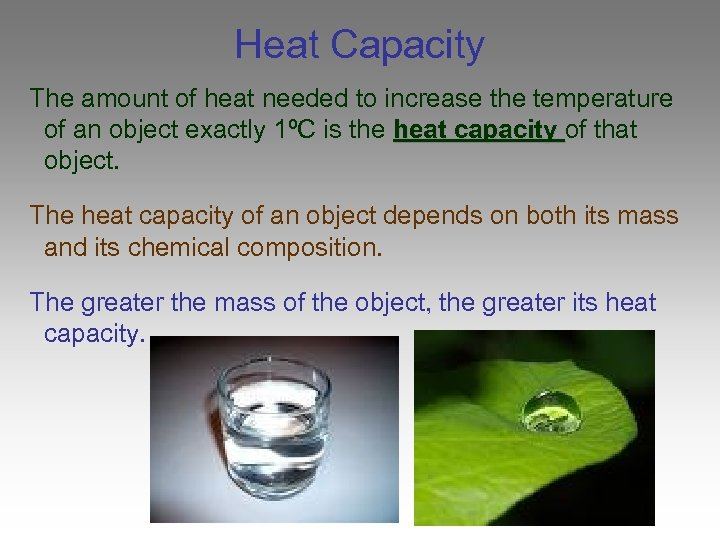Heat Capacity The amount of heat needed to increase the temperature of an object exactly 1ºC is the heat capacity of that object. The heat capacity of an object depends on both its mass and its chemical composition. The greater the mass of the object, the greater its heat capacity.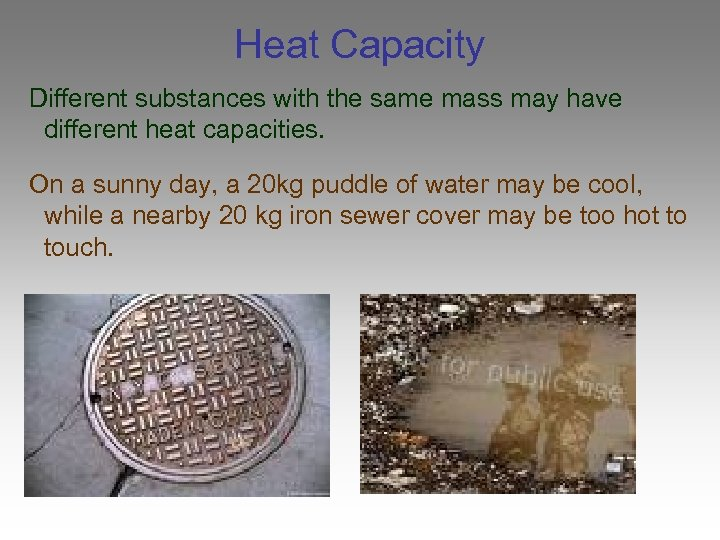Heat Capacity Different substances with the same mass may have different heat capacities. On a sunny day, a 20 kg puddle of water may be cool, while a nearby 20 kg iron sewer cover may be too hot to touch.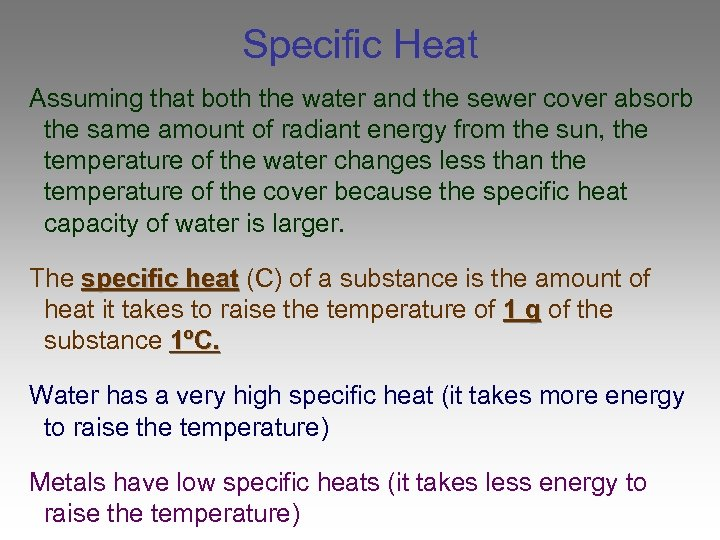Specific Heat Assuming that both the water and the sewer cover absorb the same amount of radiant energy from the sun, the temperature of the water changes less than the temperature of the cover because the specific heat capacity of water is larger. The specific heat (C) of a substance is the amount of heat it takes to raise the temperature of 1 g of the substance 1ºC. Water has a very high specific heat (it takes more energy to raise the temperature) Metals have low specific heats (it takes less energy to raise the temperature)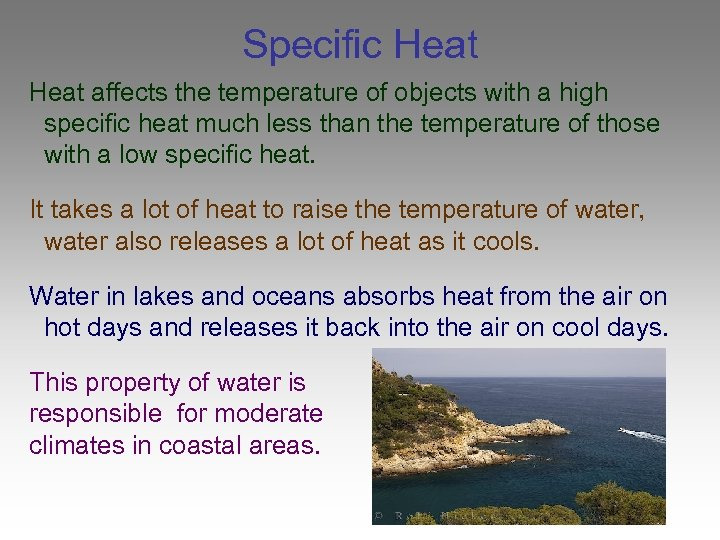Specific Heat affects the temperature of objects with a high specific heat much less than the temperature of those with a low specific heat. It takes a lot of heat to raise the temperature of water, water also releases a lot of heat as it cools. Water in lakes and oceans absorbs heat from the air on hot days and releases it back into the air on cool days. This property of water is responsible for moderate climates in coastal areas.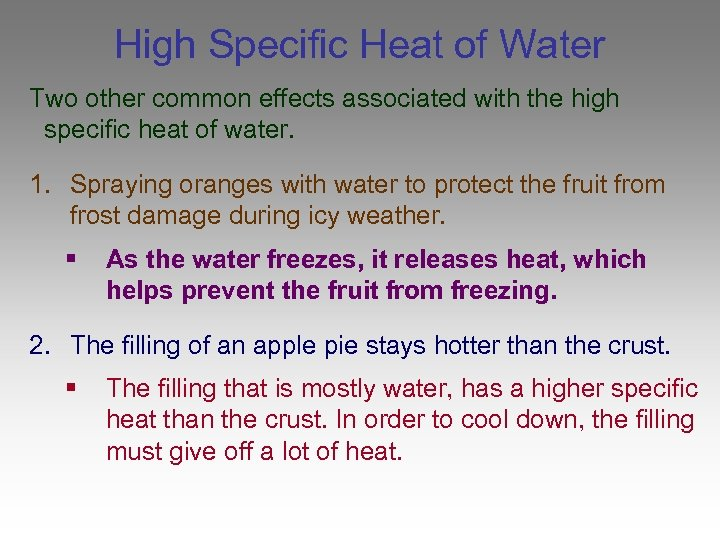High Specific Heat of Water Two other common effects associated with the high specific heat of water. 1. Spraying oranges with water to protect the fruit from frost damage during icy weather. § As the water freezes, it releases heat, which helps prevent the fruit from freezing. 2. The filling of an apple pie stays hotter than the crust. § The filling that is mostly water, has a higher specific heat than the crust. In order to cool down, the filling must give off a lot of heat.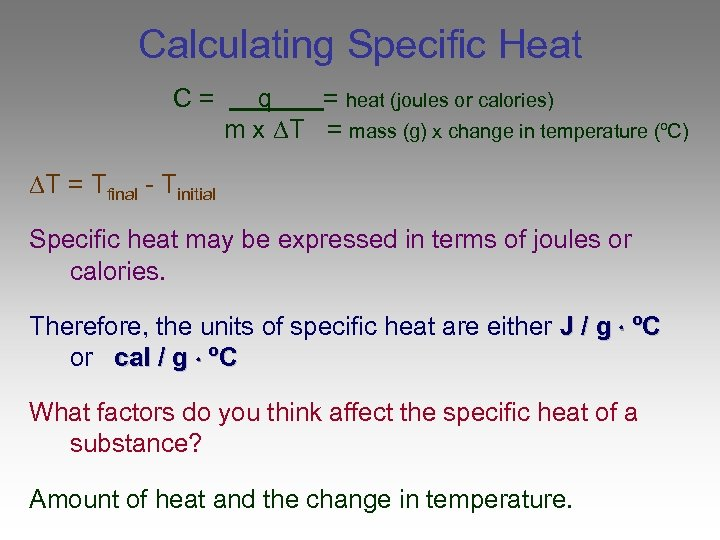Calculating Specific Heat C= q = heat (joules or calories) m x T = mass (g) x change in temperature (ºC) T = Tfinal - Tinitial Specific heat may be expressed in terms of joules or calories. Therefore, the units of specific heat are either J / g ºC or cal / g ºC What factors do you think affect the specific heat of a substance? Amount of heat and the change in temperature.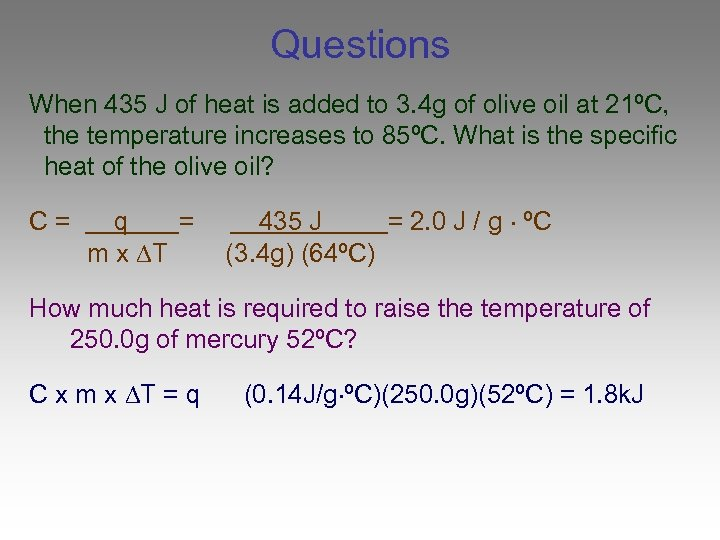Questions When 435 J of heat is added to 3. 4 g of olive oil at 21ºC, the temperature increases to 85ºC. What is the specific heat of the olive oil? C= q = m x T 435 J = 2. 0 J / g ºC (3. 4 g) (64ºC) How much heat is required to raise the temperature of 250. 0 g of mercury 52ºC? C x m x T = q (0. 14 J/g ºC)(250. 0 g)(52ºC) = 1. 8 k. J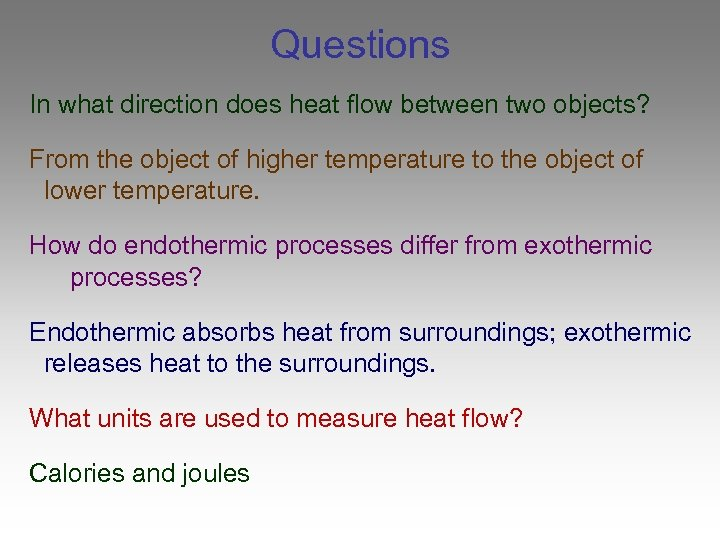Questions In what direction does heat flow between two objects? From the object of higher temperature to the object of lower temperature. How do endothermic processes differ from exothermic processes? Endothermic absorbs heat from surroundings; exothermic releases heat to the surroundings. What units are used to measure heat flow? Calories and joules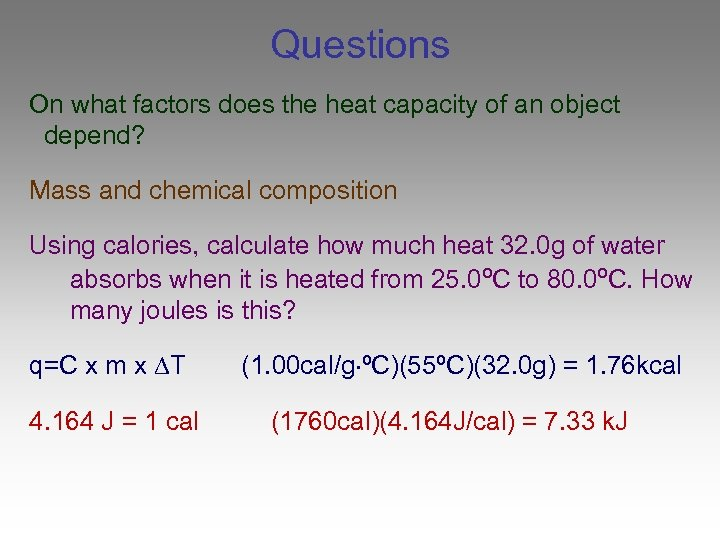Questions On what factors does the heat capacity of an object depend? Mass and chemical composition Using calories, calculate how much heat 32. 0 g of water absorbs when it is heated from 25. 0ºC to 80. 0ºC. How many joules is this? q=C x m x T 4. 164 J = 1 cal (1. 00 cal/g ºC)(55ºC)(32. 0 g) = 1. 76 kcal (1760 cal)(4. 164 J/cal) = 7. 33 k. J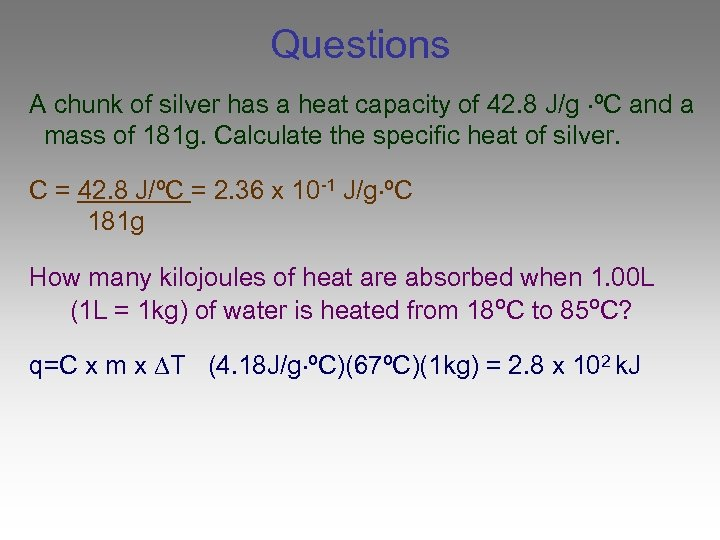Questions A chunk of silver has a heat capacity of 42. 8 J/g ºC and a mass of 181 g. Calculate the specific heat of silver. C = 42. 8 J/ºC = 2. 36 x 10 -1 J/g ºC 181 g How many kilojoules of heat are absorbed when 1. 00 L (1 L = 1 kg) of water is heated from 18ºC to 85ºC? q=C x m x T (4. 18 J/g ºC)(67ºC)(1 kg) = 2. 8 x 102 k. JEnd of Section 17. 1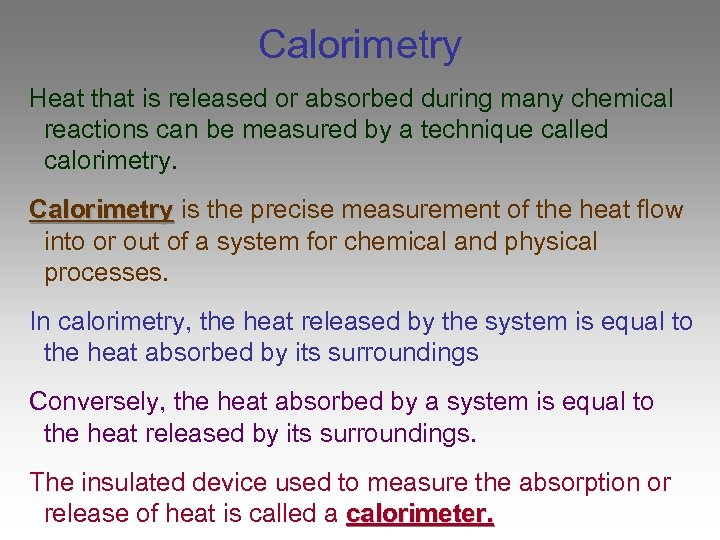Calorimetry Heat that is released or absorbed during many chemical reactions can be measured by a technique called calorimetry. Calorimetry is the precise measurement of the heat flow into or out of a system for chemical and physical processes. In calorimetry, the heat released by the system is equal to the heat absorbed by its surroundings Conversely, the heat absorbed by a system is equal to the heat released by its surroundings. The insulated device used to measure the absorption or release of heat is called a calorimeter.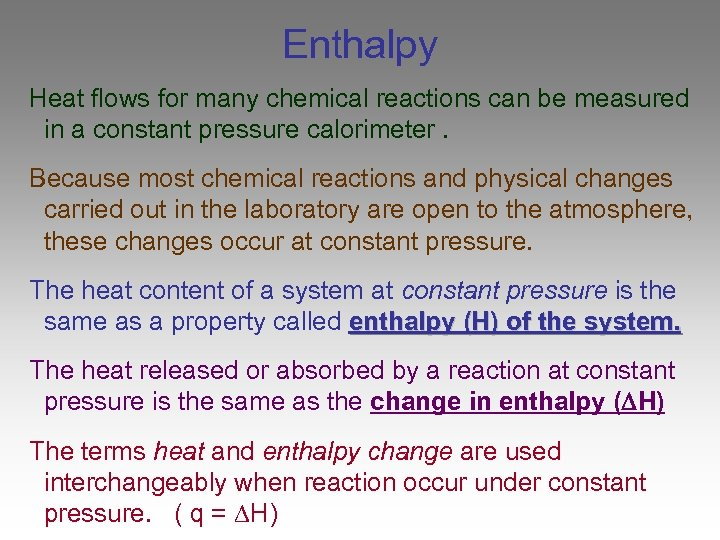Enthalpy Heat flows for many chemical reactions can be measured in a constant pressure calorimeter. Because most chemical reactions and physical changes carried out in the laboratory are open to the atmosphere, these changes occur at constant pressure. The heat content of a system at constant pressure is the same as a property called enthalpy (H) of the system. The heat released or absorbed by a reaction at constant pressure is the same as the change in enthalpy ( H) The terms heat and enthalpy change are used interchangeably when reaction occur under constant pressure. ( q = H)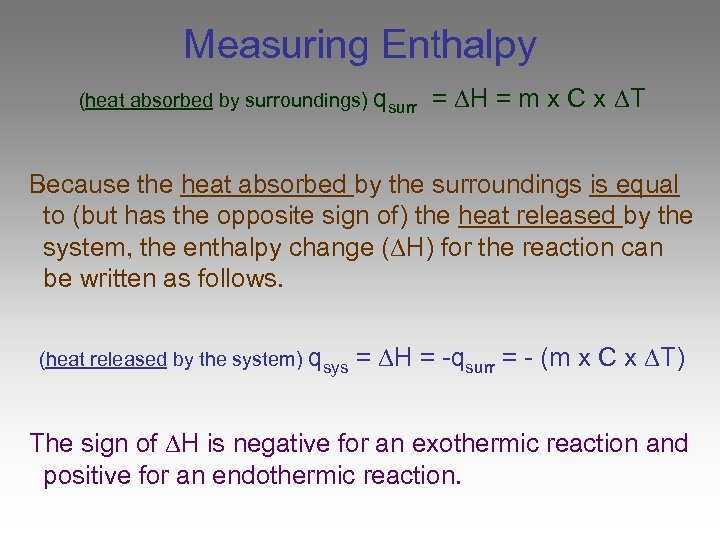Measuring Enthalpy (heat absorbed by surroundings) qsurr = H = m x C x T Because the heat absorbed by the surroundings is equal to (but has the opposite sign of) the heat released by the system, the enthalpy change ( H) for the reaction can be written as follows. (heat released by the system) qsys = H = -qsurr = - (m x C x T) The sign of H is negative for an exothermic reaction and positive for an endothermic reaction.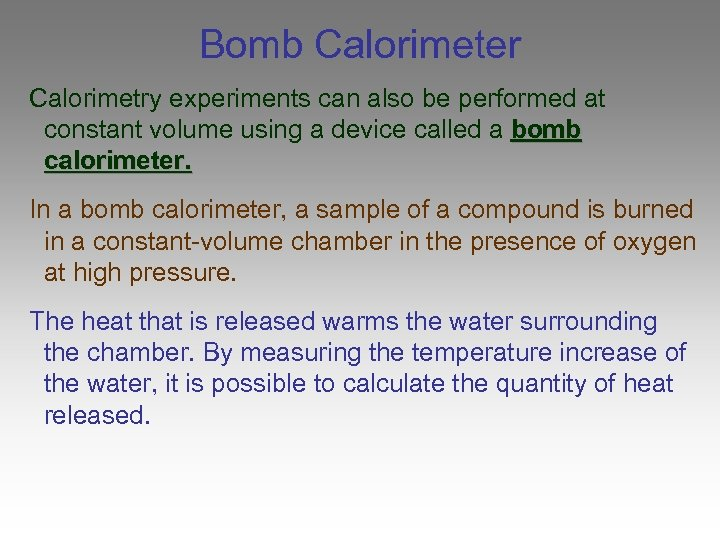Bomb Calorimeter Calorimetry experiments can also be performed at constant volume using a device called a bomb calorimeter. In a bomb calorimeter, a sample of a compound is burned in a constant-volume chamber in the presence of oxygen at high pressure. The heat that is released warms the water surrounding the chamber. By measuring the temperature increase of the water, it is possible to calculate the quantity of heat released.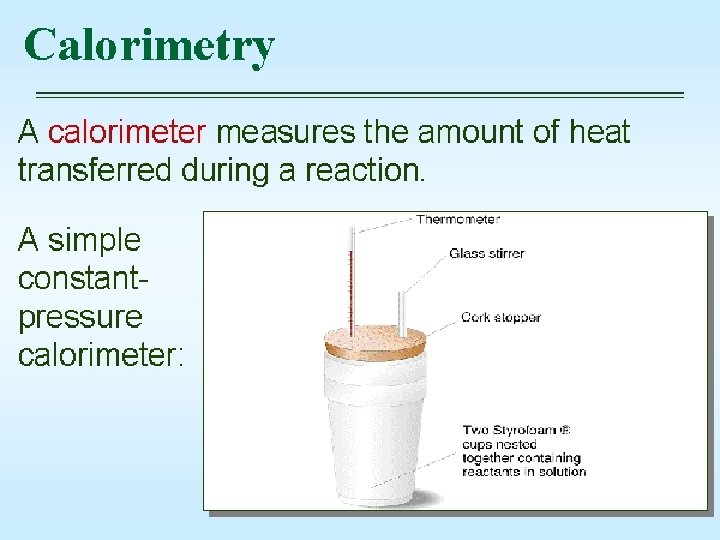Foam Cup Calorimeter (Constant pressure)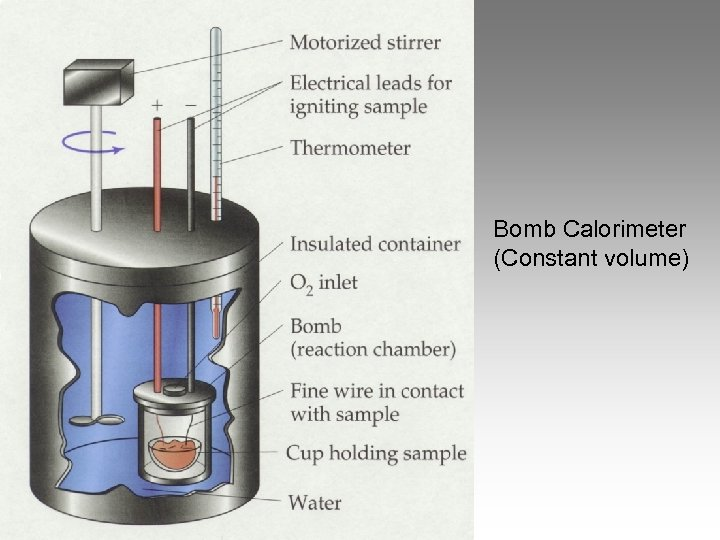Bomb Calorimeter (Constant volume)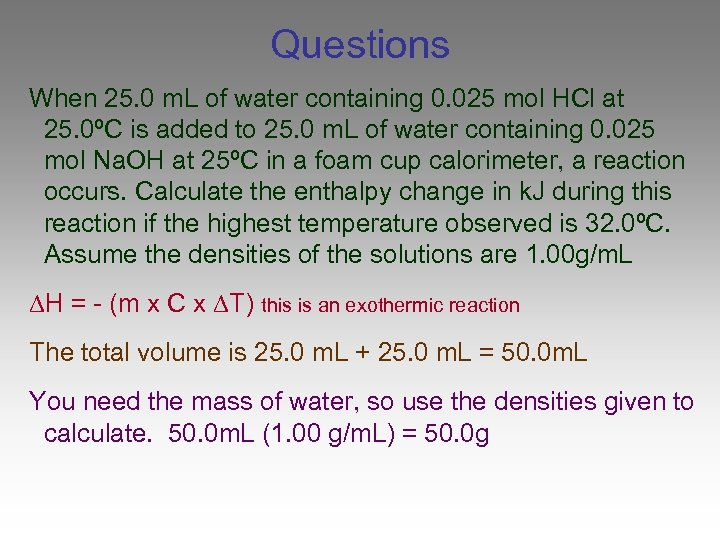Questions When 25. 0 m. L of water containing 0. 025 mol HCl at 25. 0ºC is added to 25. 0 m. L of water containing 0. 025 mol Na. OH at 25ºC in a foam cup calorimeter, a reaction occurs. Calculate the enthalpy change in k. J during this reaction if the highest temperature observed is 32. 0ºC. Assume the densities of the solutions are 1. 00 g/m. L H = - (m x C x T) this is an exothermic reaction The total volume is 25. 0 m. L + 25. 0 m. L = 50. 0 m. L You need the mass of water, so use the densities given to calculate. 50. 0 m. L (1. 00 g/m. L) = 50. 0 g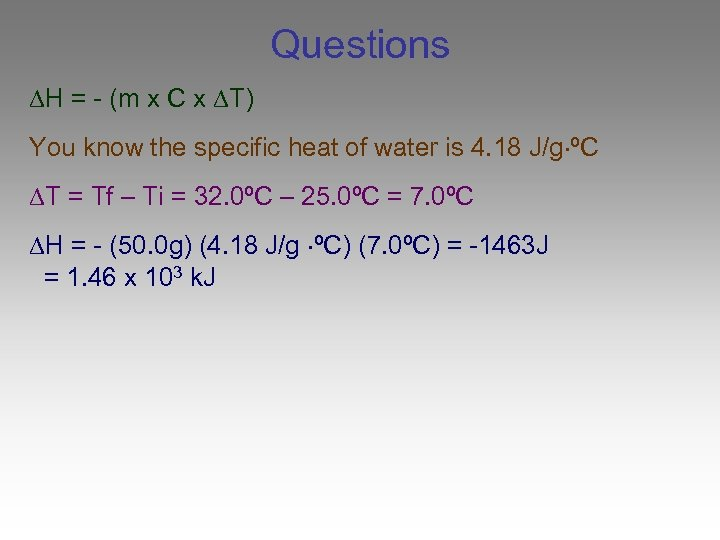Questions H = - (m x C x T) You know the specific heat of water is 4. 18 J/g ºC T = Tf – Ti = 32. 0ºC – 25. 0ºC = 7. 0ºC H = - (50. 0 g) (4. 18 J/g ºC) (7. 0ºC) = -1463 J = 1. 46 x 103 k. J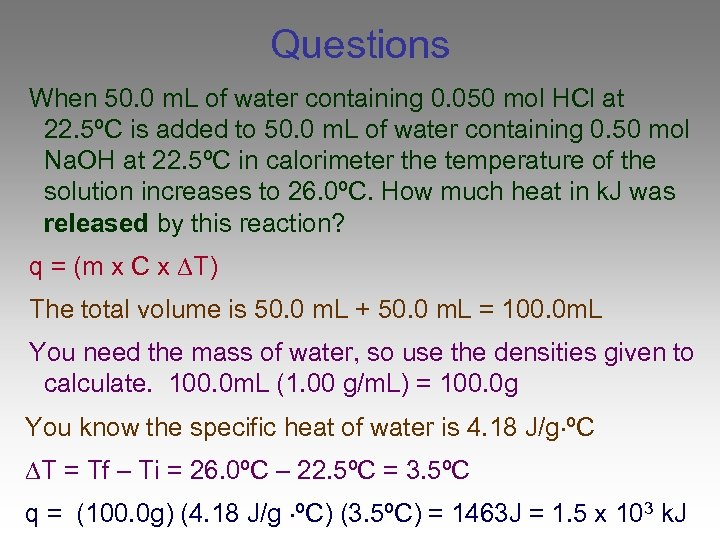Questions When 50. 0 m. L of water containing 0. 050 mol HCl at 22. 5ºC is added to 50. 0 m. L of water containing 0. 50 mol Na. OH at 22. 5ºC in calorimeter the temperature of the solution increases to 26. 0ºC. How much heat in k. J was released by this reaction? q = (m x C x T) The total volume is 50. 0 m. L + 50. 0 m. L = 100. 0 m. L You need the mass of water, so use the densities given to calculate. 100. 0 m. L (1. 00 g/m. L) = 100. 0 g You know the specific heat of water is 4. 18 J/g ºC T = Tf – Ti = 26. 0ºC – 22. 5ºC = 3. 5ºC q = (100. 0 g) (4. 18 J/g ºC) (3. 5ºC) = 1463 J = 1. 5 x 103 k. J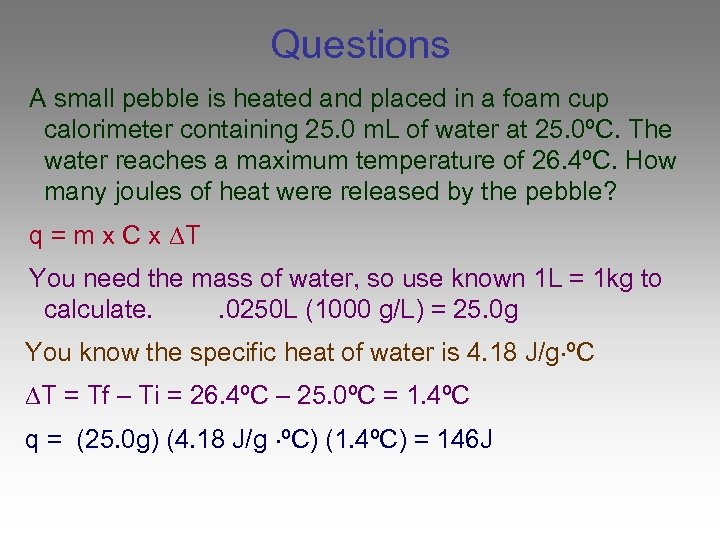Questions A small pebble is heated and placed in a foam cup calorimeter containing 25. 0 m. L of water at 25. 0ºC. The water reaches a maximum temperature of 26. 4ºC. How many joules of heat were released by the pebble? q = m x C x T You need the mass of water, so use known 1 L = 1 kg to calculate. . 0250 L (1000 g/L) = 25. 0 g You know the specific heat of water is 4. 18 J/g ºC T = Tf – Ti = 26. 4ºC – 25. 0ºC = 1. 4ºC q = (25. 0 g) (4. 18 J/g ºC) (1. 4ºC) = 146 J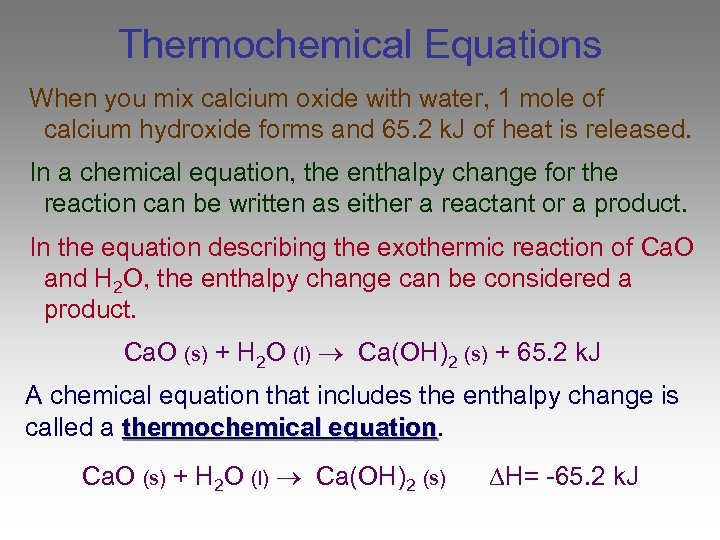Thermochemical Equations When you mix calcium oxide with water, 1 mole of calcium hydroxide forms and 65. 2 k. J of heat is released. In a chemical equation, the enthalpy change for the reaction can be written as either a reactant or a product. In the equation describing the exothermic reaction of Ca. O and H 2 O, the enthalpy change can be considered a product. Ca. O (s) + H 2 O (l) Ca(OH)2 (s) + 65. 2 k. J A chemical equation that includes the enthalpy change is called a thermochemical equation Ca. O (s) + H 2 O (l) Ca(OH)2 (s) H= -65. 2 k. J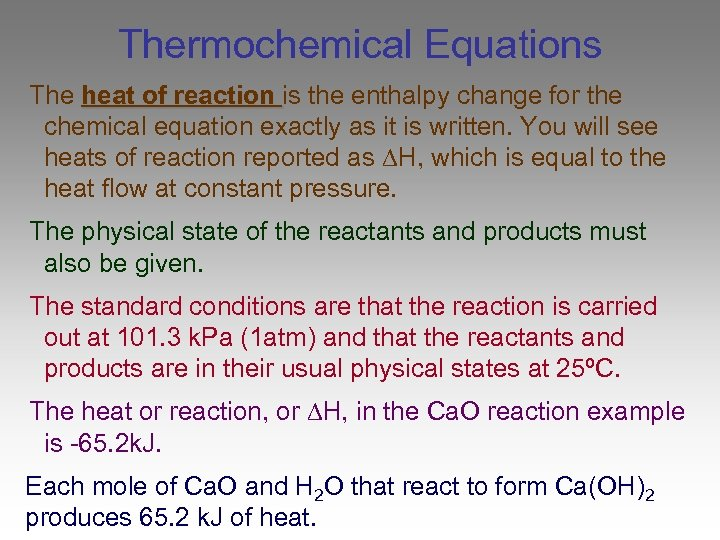Thermochemical Equations The heat of reaction is the enthalpy change for the chemical equation exactly as it is written. You will see heats of reaction reported as H, which is equal to the heat flow at constant pressure. The physical state of the reactants and products must also be given. The standard conditions are that the reaction is carried out at 101. 3 k. Pa (1 atm) and that the reactants and products are in their usual physical states at 25ºC. The heat or reaction, or H, in the Ca. O reaction example is -65. 2 k. J. Each mole of Ca. O and H 2 O that react to form Ca(OH)2 produces 65. 2 k. J of heat.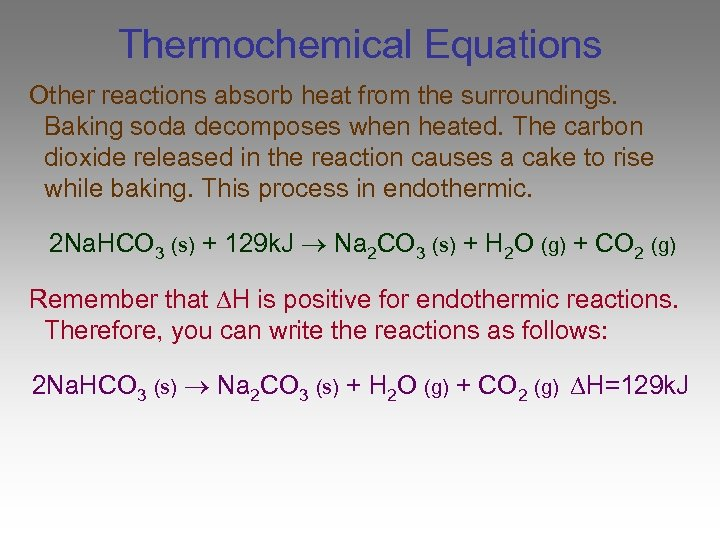Thermochemical Equations Other reactions absorb heat from the surroundings. Baking soda decomposes when heated. The carbon dioxide released in the reaction causes a cake to rise while baking. This process in endothermic. 2 Na. HCO 3 (s) + 129 k. J Na 2 CO 3 (s) + H 2 O (g) + CO 2 (g) Remember that H is positive for endothermic reactions. Therefore, you can write the reactions as follows: 2 Na. HCO 3 (s) Na 2 CO 3 (s) + H 2 O (g) + CO 2 (g) H=129 k. J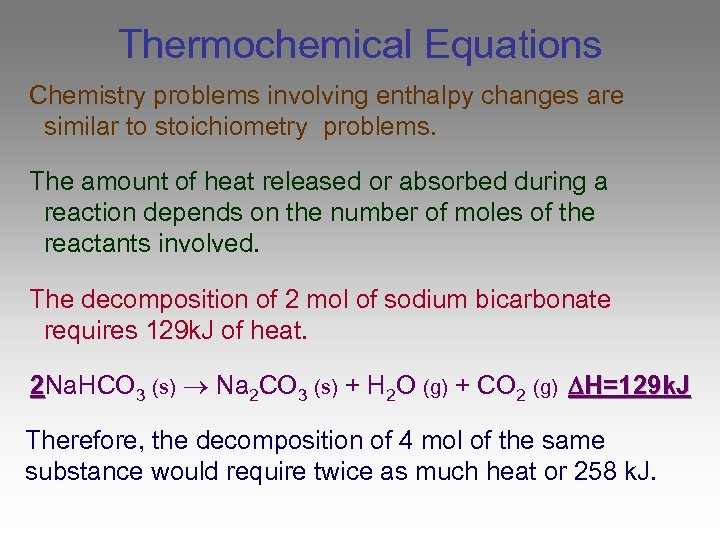Thermochemical Equations Chemistry problems involving enthalpy changes are similar to stoichiometry problems. The amount of heat released or absorbed during a reaction depends on the number of moles of the reactants involved. The decomposition of 2 mol of sodium bicarbonate requires 129 k. J of heat. 2 Na. HCO 3 (s) Na 2 CO 3 (s) + H 2 O (g) + CO 2 (g) H=129 k. J Therefore, the decomposition of 4 mol of the same substance would require twice as much heat or 258 k. J.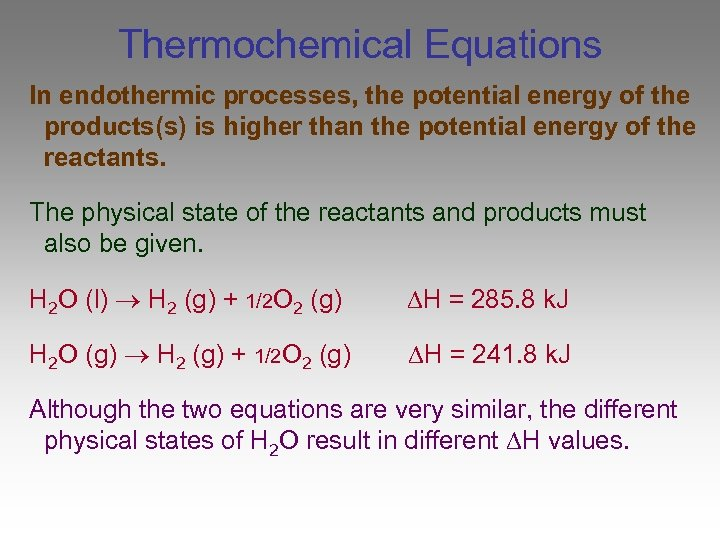Thermochemical Equations In endothermic processes, the potential energy of the products(s) is higher than the potential energy of the reactants. The physical state of the reactants and products must also be given. H 2 O (l) H 2 (g) + 1/2 O 2 (g) H = 285. 8 k. J H 2 O (g) H 2 (g) + 1/2 O 2 (g) H = 241. 8 k. J Although the two equations are very similar, the different physical states of H 2 O result in different H values.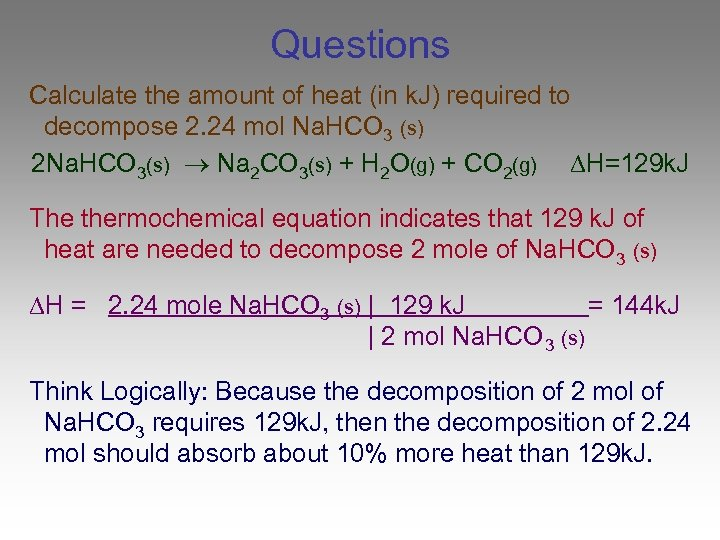Questions Calculate the amount of heat (in k. J) required to decompose 2. 24 mol Na. HCO 3 (s) 2 Na. HCO 3(s) Na 2 CO 3(s) + H 2 O(g) + CO 2(g) H=129 k. J The thermochemical equation indicates that 129 k. J of heat are needed to decompose 2 mole of Na. HCO 3 (s) H = 2. 24 mole Na. HCO 3 (s) | 129 k. J = 144 k. J | 2 mol Na. HCO 3 (s) Think Logically: Because the decomposition of 2 mol of Na. HCO 3 requires 129 k. J, then the decomposition of 2. 24 mol should absorb about 10% more heat than 129 k. J.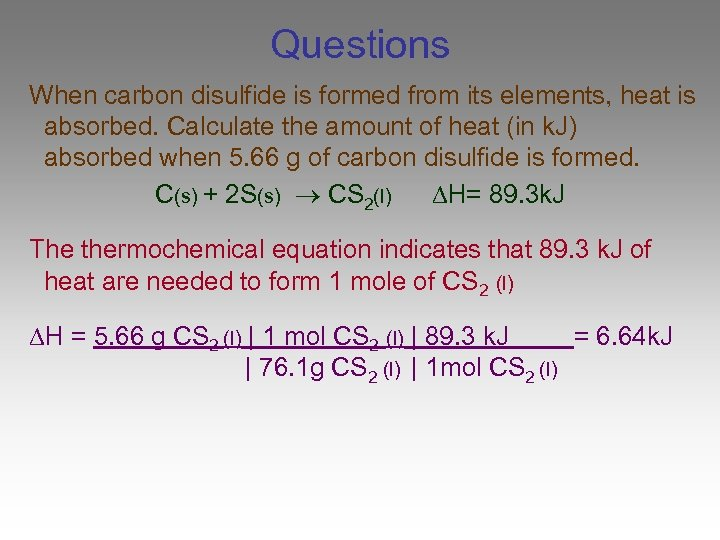Questions When carbon disulfide is formed from its elements, heat is absorbed. Calculate the amount of heat (in k. J) absorbed when 5. 66 g of carbon disulfide is formed. C(s) + 2 S(s) CS 2(l) H= 89. 3 k. J The thermochemical equation indicates that 89. 3 k. J of heat are needed to form 1 mole of CS 2 (l) H = 5. 66 g CS 2 (l) | 1 mol CS 2 (l) | 89. 3 k. J = 6. 64 k. J | 76. 1 g CS 2 (l) | 1 mol CS 2 (l)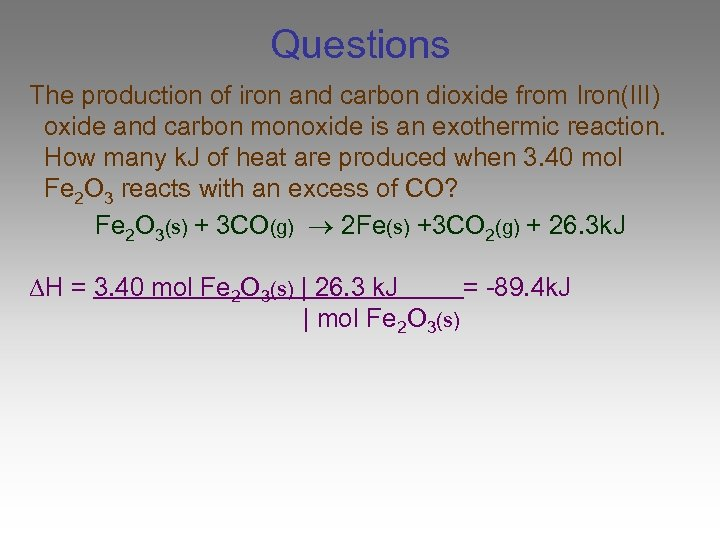Questions The production of iron and carbon dioxide from Iron(III) oxide and carbon monoxide is an exothermic reaction. How many k. J of heat are produced when 3. 40 mol Fe 2 O 3 reacts with an excess of CO? Fe 2 O 3(s) + 3 CO(g) 2 Fe(s) +3 CO 2(g) + 26. 3 k. J H = 3. 40 mol Fe 2 O 3(s) | 26. 3 k. J = -89. 4 k. J | mol Fe 2 O 3(s)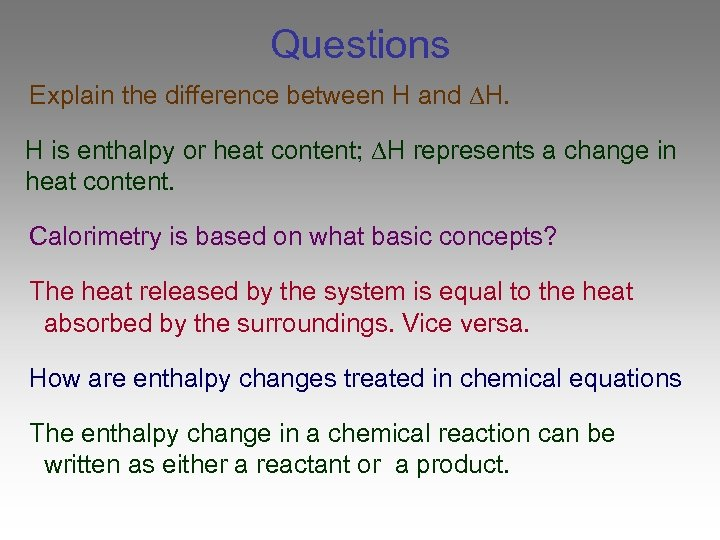Questions Explain the difference between H and H. H is enthalpy or heat content; H represents a change in heat content. Calorimetry is based on what basic concepts? The heat released by the system is equal to the heat absorbed by the surroundings. Vice versa. How are enthalpy changes treated in chemical equations The enthalpy change in a chemical reaction can be written as either a reactant or a product.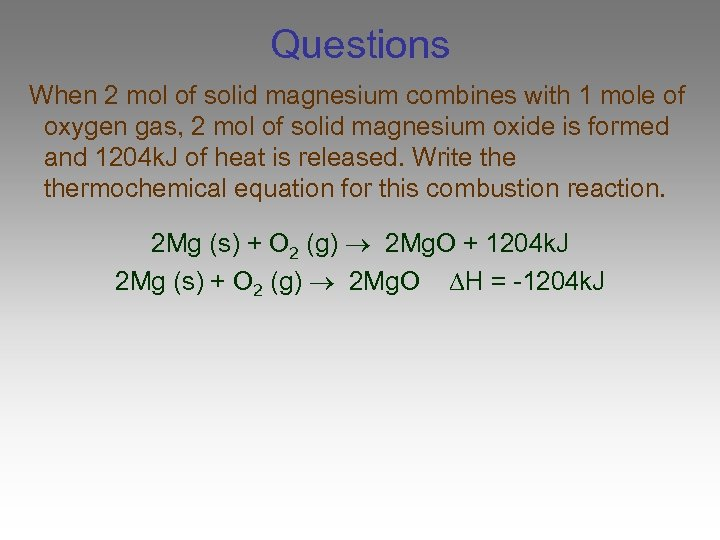Questions When 2 mol of solid magnesium combines with 1 mole of oxygen gas, 2 mol of solid magnesium oxide is formed and 1204 k. J of heat is released. Write thermochemical equation for this combustion reaction. 2 Mg (s) + O 2 (g) 2 Mg. O + 1204 k. J 2 Mg (s) + O 2 (g) 2 Mg. O H = -1204 k. J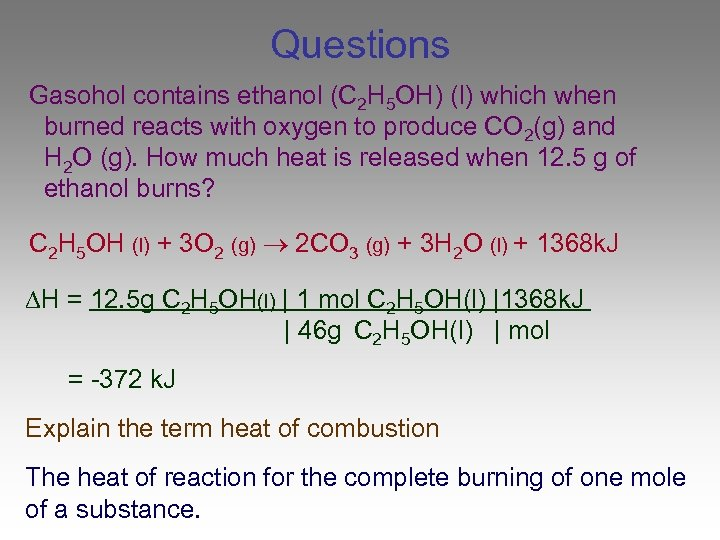Questions Gasohol contains ethanol (C 2 H 5 OH) (l) which when burned reacts with oxygen to produce CO 2(g) and H 2 O (g). How much heat is released when 12. 5 g of ethanol burns? C 2 H 5 OH (l) + 3 O 2 (g) 2 CO 3 (g) + 3 H 2 O (l) + 1368 k. J H = 12. 5 g C 2 H 5 OH(l) | 1 mol C 2 H 5 OH(l) |1368 k. J | 46 g C 2 H 5 OH(l) | mol = -372 k. J Explain the term heat of combustion The heat of reaction for the complete burning of one mole of a substance.End of Section 17. 2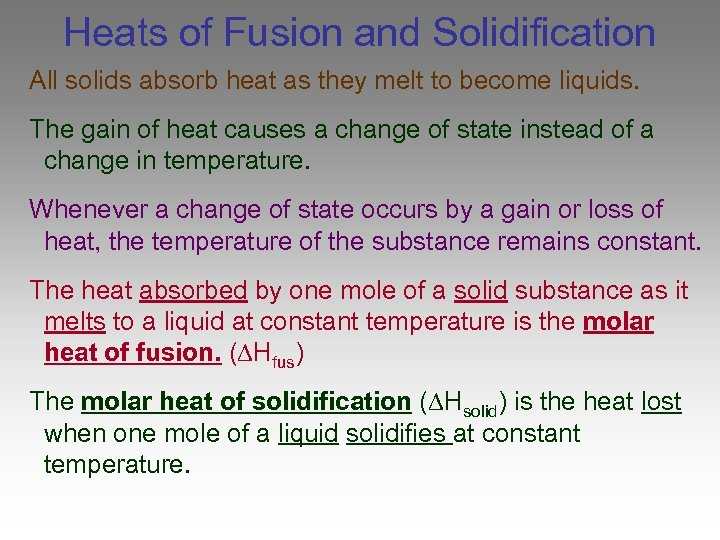Heats of Fusion and Solidification All solids absorb heat as they melt to become liquids. The gain of heat causes a change of state instead of a change in temperature. Whenever a change of state occurs by a gain or loss of heat, the temperature of the substance remains constant. The heat absorbed by one mole of a solid substance as it melts to a liquid at constant temperature is the molar heat of fusion. ( Hfus) The molar heat of solidification ( Hsolid) is the heat lost when one mole of a liquid solidifies at constant temperature.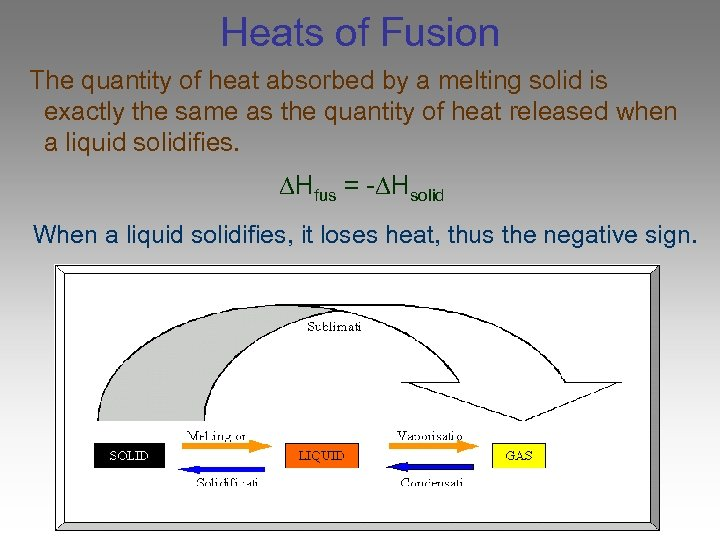Heats of Fusion The quantity of heat absorbed by a melting solid is exactly the same as the quantity of heat released when a liquid solidifies. Hfus = - Hsolid When a liquid solidifies, it loses heat, thus the negative sign.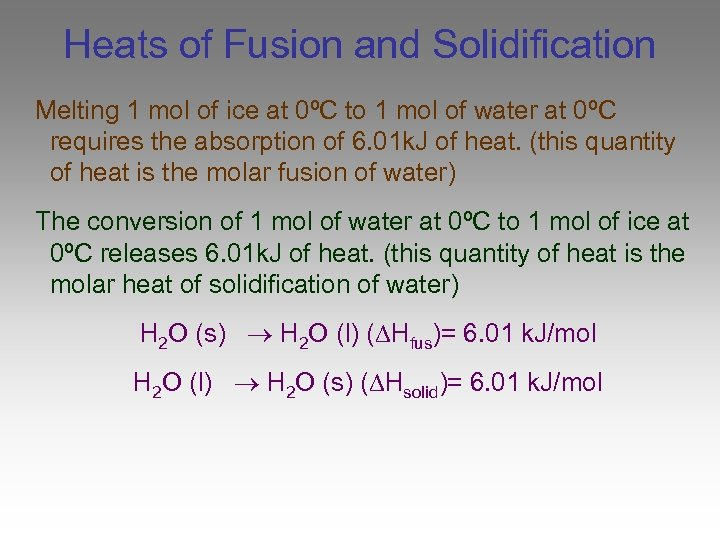Heats of Fusion and Solidification Melting 1 mol of ice at 0ºC to 1 mol of water at 0ºC requires the absorption of 6. 01 k. J of heat. (this quantity of heat is the molar fusion of water) The conversion of 1 mol of water at 0ºC to 1 mol of ice at 0ºC releases 6. 01 k. J of heat. (this quantity of heat is the molar heat of solidification of water) H 2 O (s) H 2 O (l) ( Hfus)= 6. 01 k. J/mol H 2 O (l) H 2 O (s) ( Hsolid)= 6. 01 k. J/mol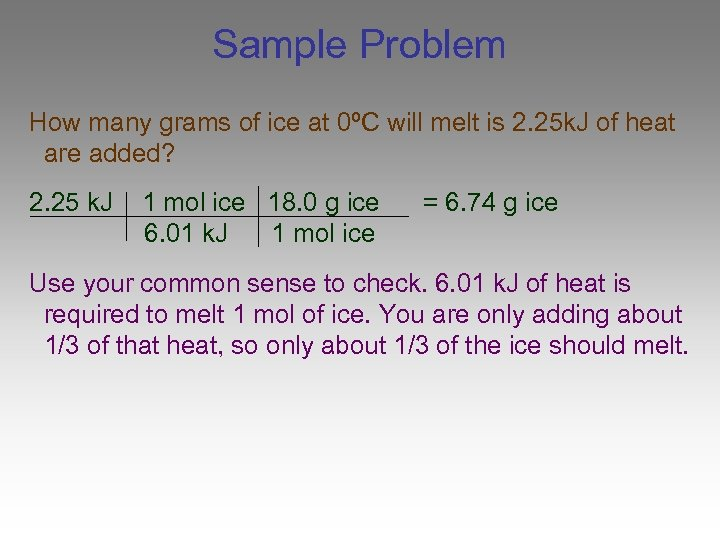Sample Problem How many grams of ice at 0ºC will melt is 2. 25 k. J of heat are added? 2. 25 k. J 1 mol ice 18. 0 g ice 6. 01 k. J 1 mol ice = 6. 74 g ice Use your common sense to check. 6. 01 k. J of heat is required to melt 1 mol of ice. You are only adding about 1/3 of that heat, so only about 1/3 of the ice should melt.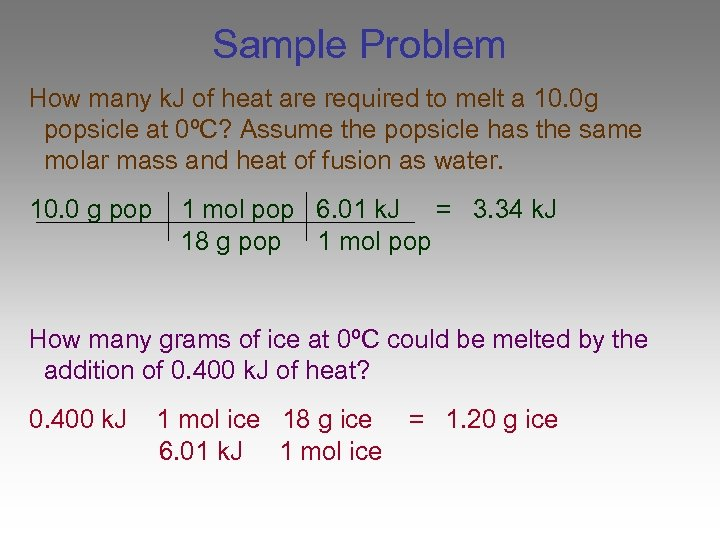Sample Problem How many k. J of heat are required to melt a 10. 0 g popsicle at 0ºC? Assume the popsicle has the same molar mass and heat of fusion as water. 10. 0 g pop 1 mol pop 6. 01 k. J = 3. 34 k. J 18 g pop 1 mol pop How many grams of ice at 0ºC could be melted by the addition of 0. 400 k. J of heat? 0. 400 k. J 1 mol ice 18 g ice 6. 01 k. J 1 mol ice = 1. 20 g ice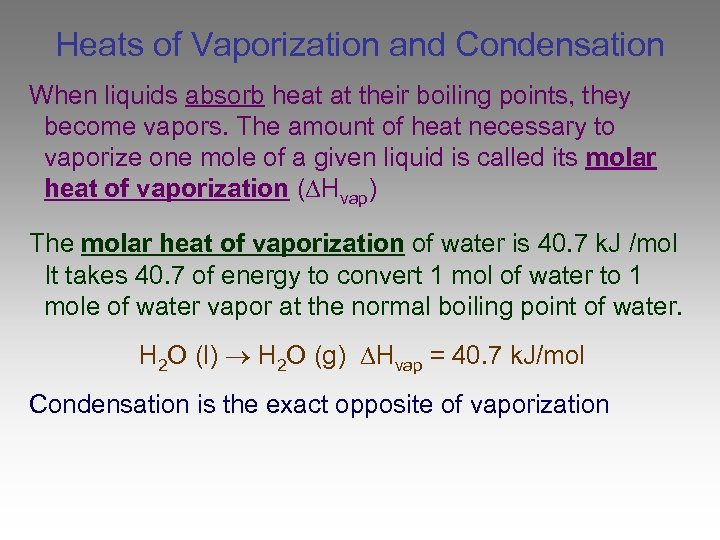Heats of Vaporization and Condensation When liquids absorb heat at their boiling points, they become vapors. The amount of heat necessary to vaporize one mole of a given liquid is called its molar heat of vaporization ( Hvap) The molar heat of vaporization of water is 40. 7 k. J /mol It takes 40. 7 of energy to convert 1 mol of water to 1 mole of water vapor at the normal boiling point of water. H 2 O (l) H 2 O (g) Hvap = 40. 7 k. J/mol Condensation is the exact opposite of vaporization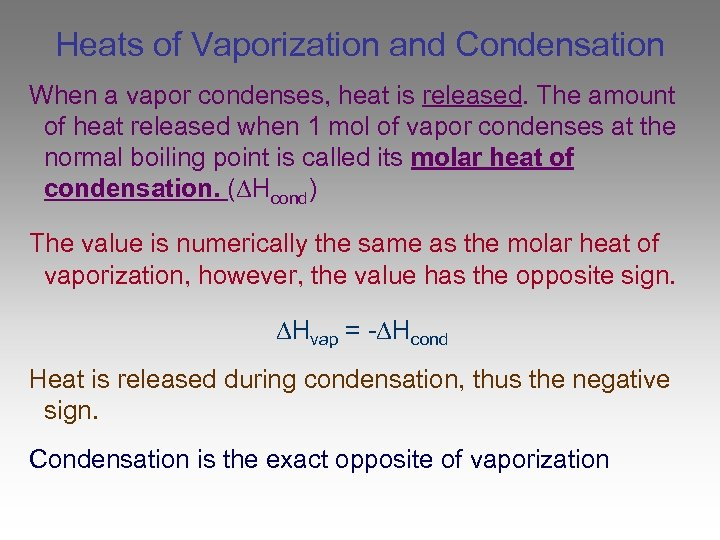Heats of Vaporization and Condensation When a vapor condenses, heat is released. The amount of heat released when 1 mol of vapor condenses at the normal boiling point is called its molar heat of condensation. ( Hcond) The value is numerically the same as the molar heat of vaporization, however, the value has the opposite sign. Hvap = - Hcond Heat is released during condensation, thus the negative sign. Condensation is the exact opposite of vaporization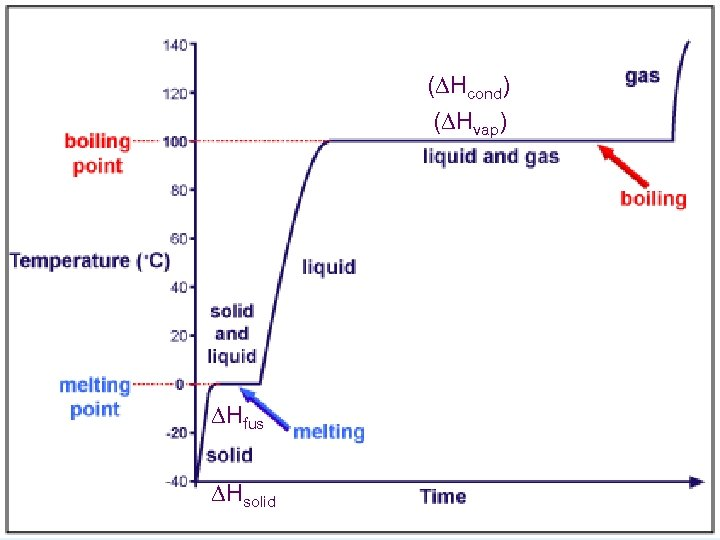( Hcond) ( Hvap) Hfus Hsolid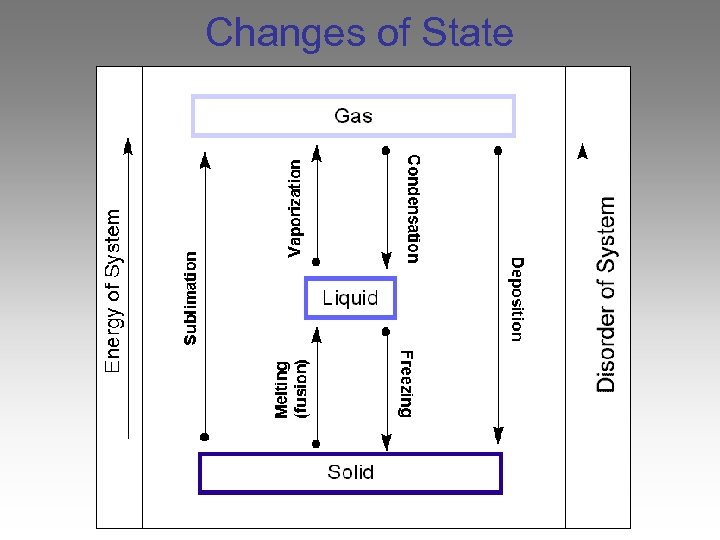Changes of State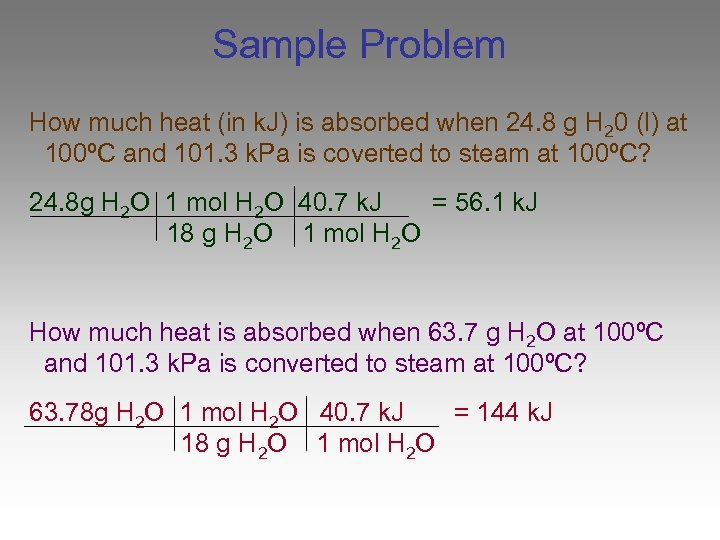Sample Problem How much heat (in k. J) is absorbed when 24. 8 g H 20 (l) at 100ºC and 101. 3 k. Pa is coverted to steam at 100ºC? 24. 8 g H 2 O 1 mol H 2 O 40. 7 k. J = 56. 1 k. J 18 g H 2 O 1 mol H 2 O How much heat is absorbed when 63. 7 g H 2 O at 100ºC and 101. 3 k. Pa is converted to steam at 100ºC? 63. 78 g H 2 O 1 mol H 2 O 40. 7 k. J = 144 k. J 18 g H 2 O 1 mol H 2 O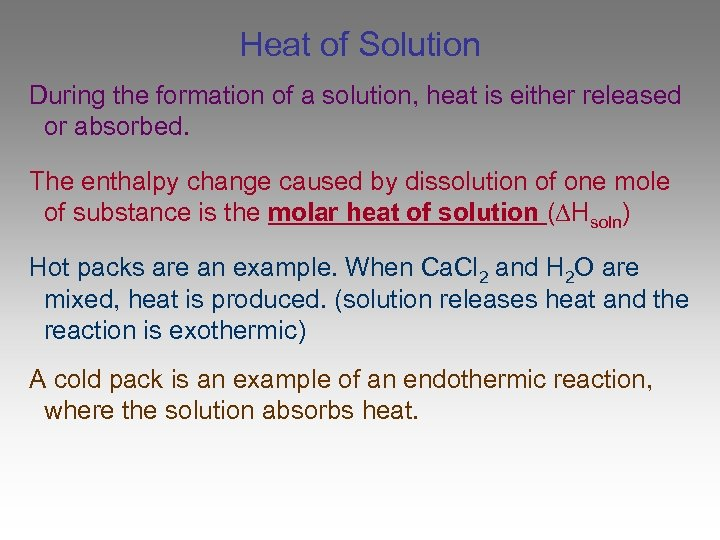Heat of Solution During the formation of a solution, heat is either released or absorbed. The enthalpy change caused by dissolution of one mole of substance is the molar heat of solution ( Hsoln) Hot packs are an example. When Ca. Cl 2 and H 2 O are mixed, heat is produced. (solution releases heat and the reaction is exothermic) A cold pack is an example of an endothermic reaction, where the solution absorbs heat.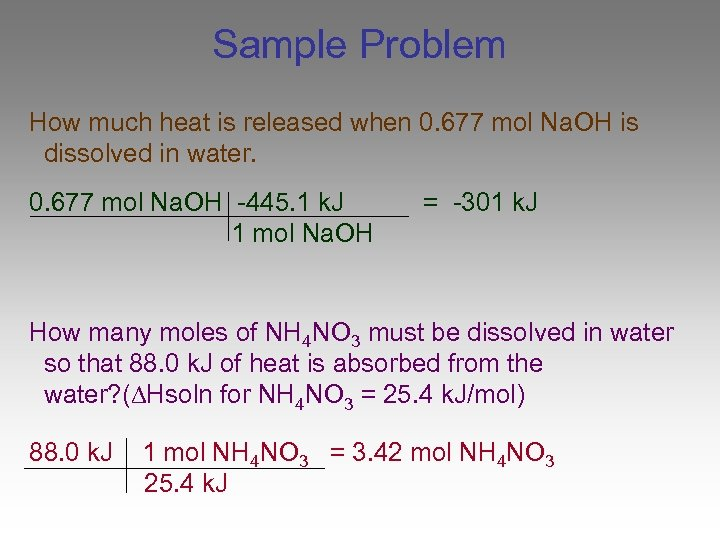Sample Problem How much heat is released when 0. 677 mol Na. OH is dissolved in water. 0. 677 mol Na. OH -445. 1 k. J 1 mol Na. OH = -301 k. J How many moles of NH 4 NO 3 must be dissolved in water so that 88. 0 k. J of heat is absorbed from the water? ( Hsoln for NH 4 NO 3 = 25. 4 k. J/mol) 88. 0 k. J 1 mol NH 4 NO 3 = 3. 42 mol NH 4 NO 3 25. 4 k. J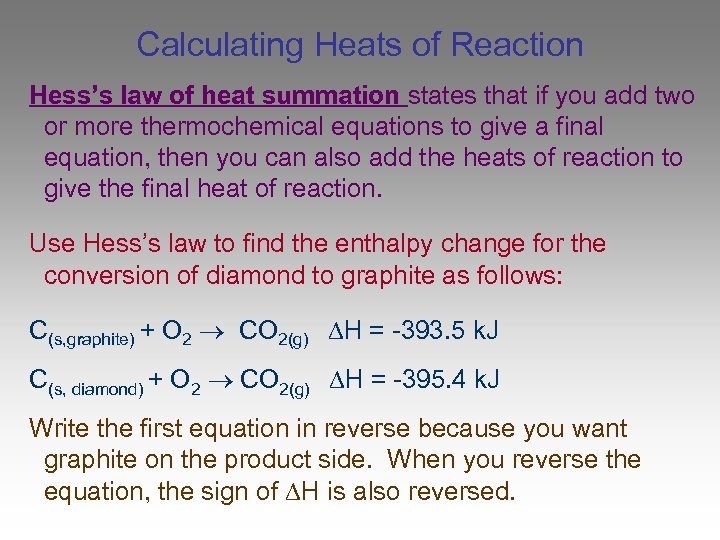Calculating Heats of Reaction Hess’s law of heat summation states that if you add two or more thermochemical equations to give a final equation, then you can also add the heats of reaction to give the final heat of reaction. Use Hess’s law to find the enthalpy change for the conversion of diamond to graphite as follows: C(s, graphite) + O 2 CO 2(g) H = -393. 5 k. J C(s, diamond) + O 2 CO 2(g) H = -395. 4 k. J Write the first equation in reverse because you want graphite on the product side. When you reverse the equation, the sign of H is also reversed.End of Section 17. 3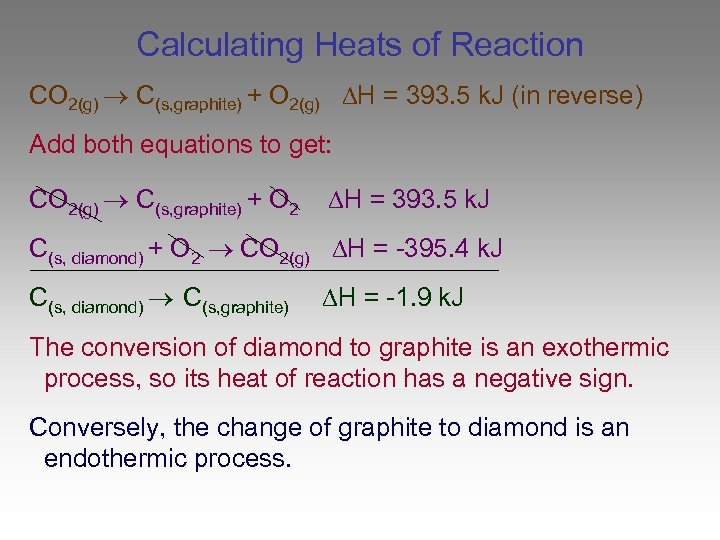Calculating Heats of Reaction CO 2(g) C(s, graphite) + O 2(g) H = 393. 5 k. J (in reverse) Add both equations to get: CO 2(g) C(s, graphite) + O 2 H = 393. 5 k. J C(s, diamond) + O 2 CO 2(g) H = -395. 4 k. J C(s, diamond) C(s, graphite) H = -1. 9 k. J The conversion of diamond to graphite is an exothermic process, so its heat of reaction has a negative sign. Conversely, the change of graphite to diamond is an endothermic process.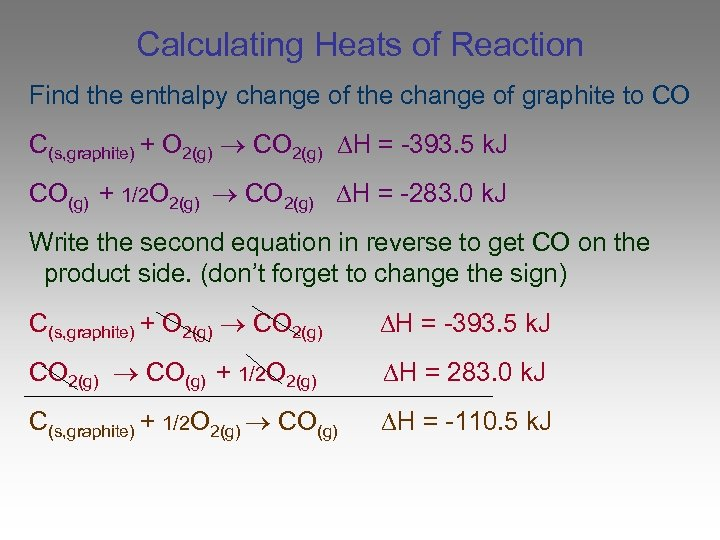Calculating Heats of Reaction Find the enthalpy change of the change of graphite to CO C(s, graphite) + O 2(g) CO 2(g) H = -393. 5 k. J CO(g) + 1/2 O 2(g) CO 2(g) H = -283. 0 k. J Write the second equation in reverse to get CO on the product side. (don’t forget to change the sign) C(s, graphite) + O 2(g) CO 2(g) H = -393. 5 k. J CO 2(g) CO(g) + 1/2 O 2(g) H = 283. 0 k. J C(s, graphite) + 1/2 O 2(g) CO(g) H = -110. 5 k. J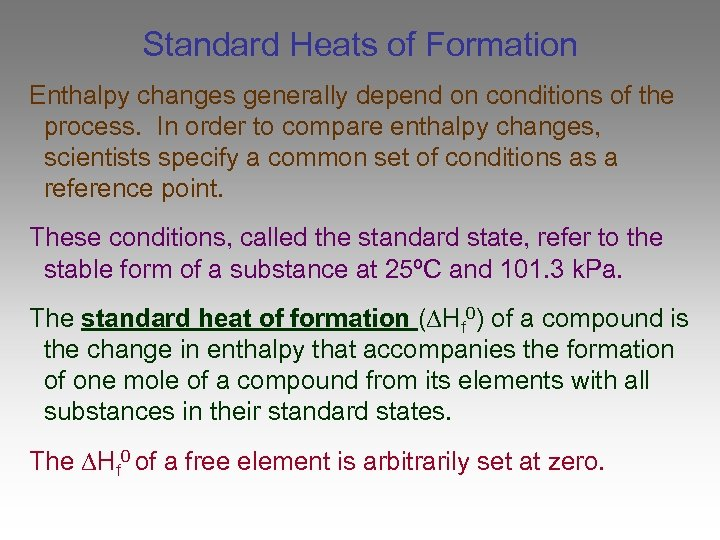Standard Heats of Formation Enthalpy changes generally depend on conditions of the process. In order to compare enthalpy changes, scientists specify a common set of conditions as a reference point. These conditions, called the standard state, refer to the stable form of a substance at 25ºC and 101. 3 k. Pa. The standard heat of formation ( Hf 0) of a compound is the change in enthalpy that accompanies the formation of one mole of a compound from its elements with all substances in their standard states. The Hf 0 of a free element is arbitrarily set at zero.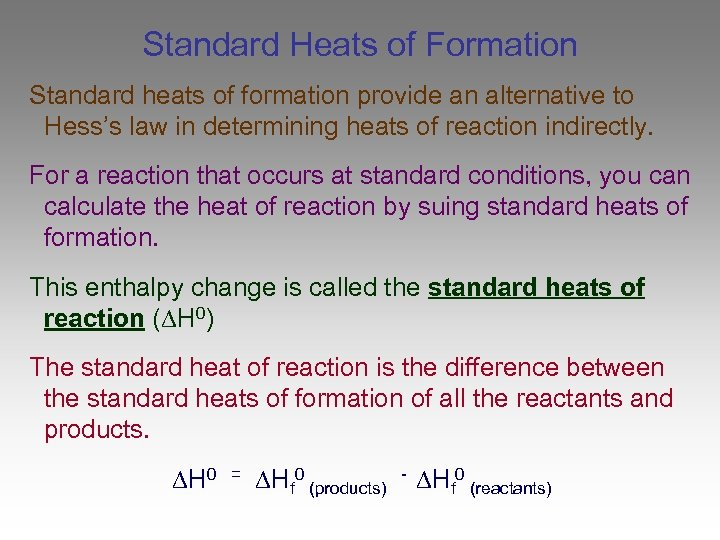Standard Heats of Formation Standard heats of formation provide an alternative to Hess’s law in determining heats of reaction indirectly. For a reaction that occurs at standard conditions, you can calculate the heat of reaction by suing standard heats of formation. This enthalpy change is called the standard heats of reaction ( H 0) The standard heat of reaction is the difference between the standard heats of formation of all the reactants and products. H 0 = Hf 0 (products) - Hf 0 (reactants)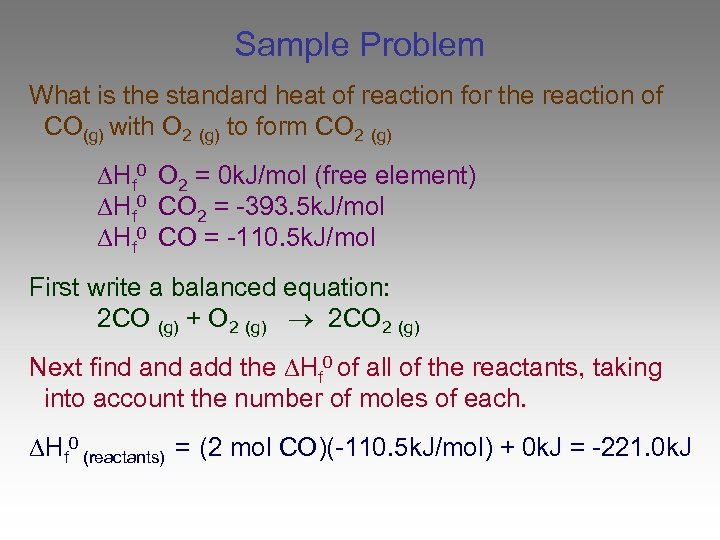Sample Problem What is the standard heat of reaction for the reaction of CO(g) with O 2 (g) to form CO 2 (g) Hf 0 O 2 = 0 k. J/mol (free element) Hf 0 CO 2 = -393. 5 k. J/mol Hf 0 CO = -110. 5 k. J/mol First write a balanced equation: 2 CO (g) + O 2 (g) 2 CO 2 (g) Next find add the Hf 0 of all of the reactants, taking into account the number of moles of each. Hf 0 (reactants) = (2 mol CO)(-110. 5 k. J/mol) + 0 k. J = -221. 0 k. J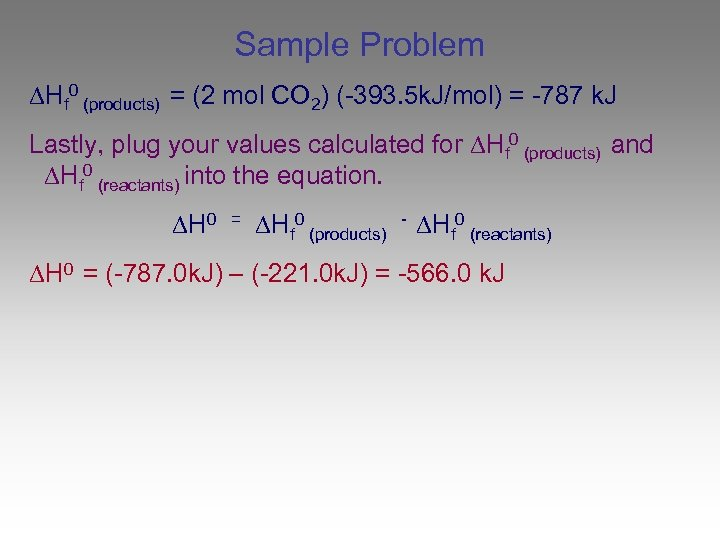Sample Problem Hf 0 (products) = (2 mol CO 2) (-393. 5 k. J/mol) = -787 k. J Lastly, plug your values calculated for Hf 0 (products) and Hf 0 (reactants) into the equation. H 0 = Hf 0 (products) - Hf 0 (reactants) H 0 = (-787. 0 k. J) – (-221. 0 k. J) = -566. 0 k. J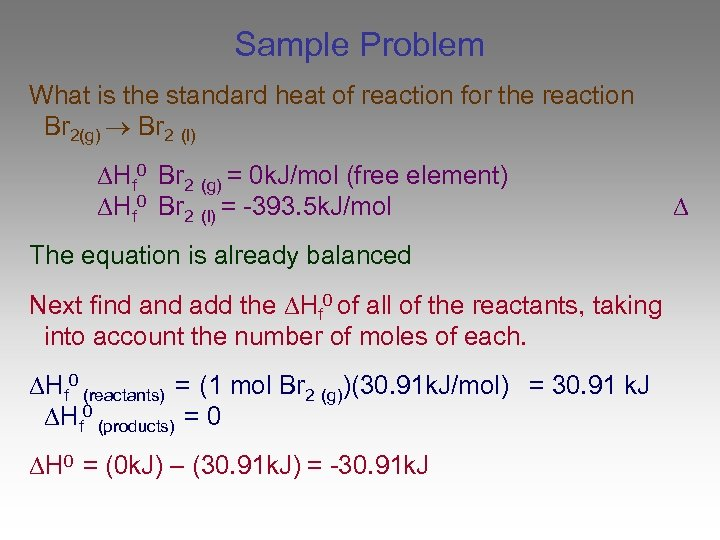Sample Problem What is the standard heat of reaction for the reaction Br 2(g) Br 2 (l) Hf 0 Br 2 (g) = 0 k. J/mol (free element) Hf 0 Br 2 (l) = -393. 5 k. J/mol The equation is already balanced Next find add the Hf 0 of all of the reactants, taking into account the number of moles of each. Hf 0 (reactants) = (1 mol Br 2 (g))(30. 91 k. J/mol) = 30. 91 k. J Hf 0 (products) = 0 H 0 = (0 k. J) – (30. 91 k. J) = -30. 91 k. J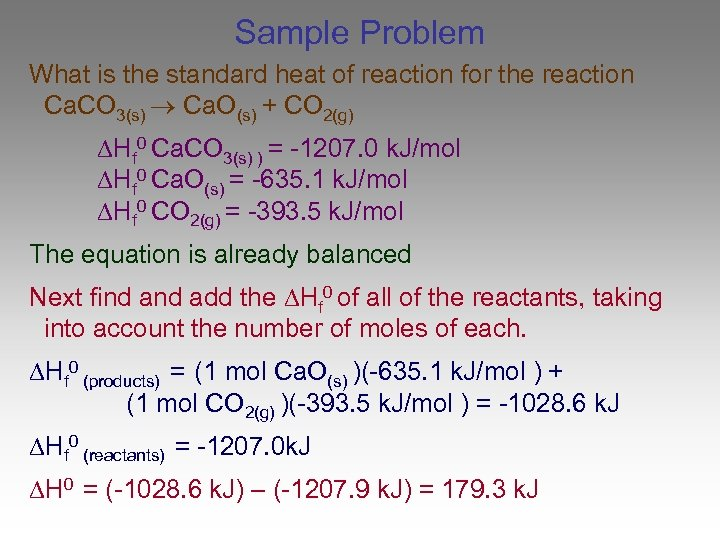Sample Problem What is the standard heat of reaction for the reaction Ca. CO 3(s) Ca. O(s) + CO 2(g) Hf 0 Ca. CO 3(s) ) = -1207. 0 k. J/mol Hf 0 Ca. O(s) = -635. 1 k. J/mol Hf 0 CO 2(g) = -393. 5 k. J/mol The equation is already balanced Next find add the Hf 0 of all of the reactants, taking into account the number of moles of each. Hf 0 (products) = (1 mol Ca. O(s) )(-635. 1 k. J/mol ) + (1 mol CO 2(g) )(-393. 5 k. J/mol ) = -1028. 6 k. J Hf 0 (reactants) = -1207. 0 k. J H 0 = (-1028. 6 k. J) – (-1207. 9 k. J) = 179. 3 k. JEnd of Chapter 17